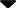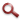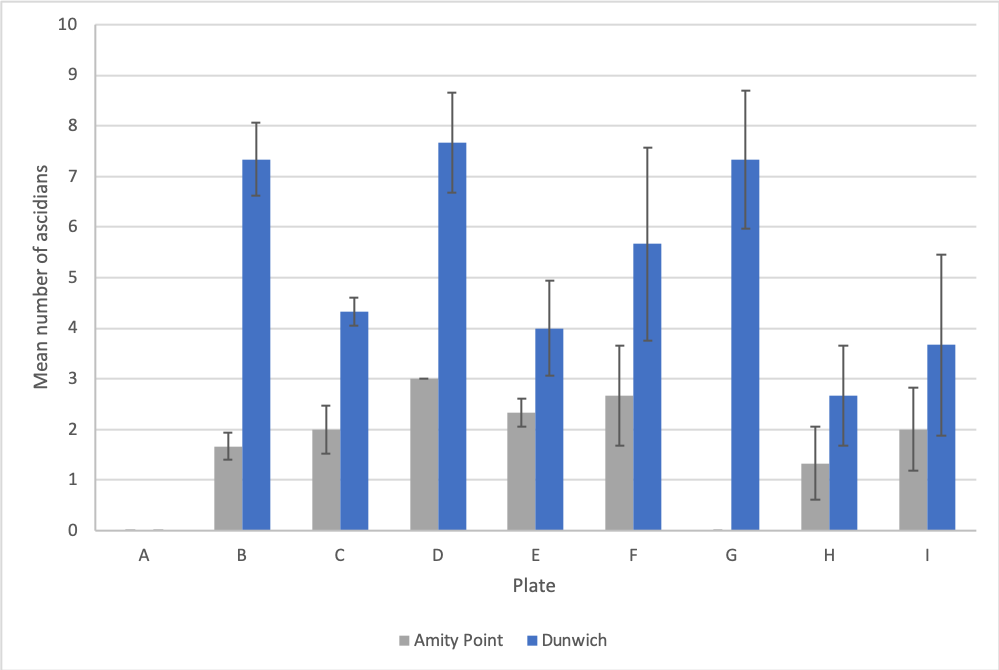Site WebYou are here:

## Student Project FiguresFigure 4 The average number of ascidians (+/-SE) counted on each plate across each ARMS in Dunwich (Blue) and Amity Point (Grey). Plate 1=A, Plate 2=B, Plate 3=C etc. In Dunwich significant relationships are apparent between plates A&B (p=0.014), A&C (p=0.005), A&D (p=0.023), A&G (p=0.047) and B&C (p=0.035) n=128. In Amity Point significance is seen between plates A&B (p=0.037), A&E (p=0.019), B&G (p=0.037) and E&G (p=0.019) n=51. Figure 4 Figure 5